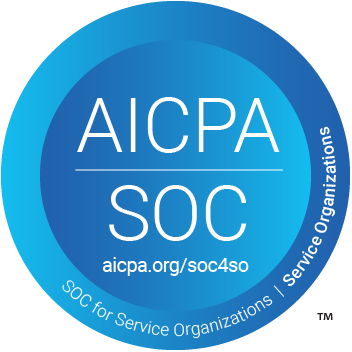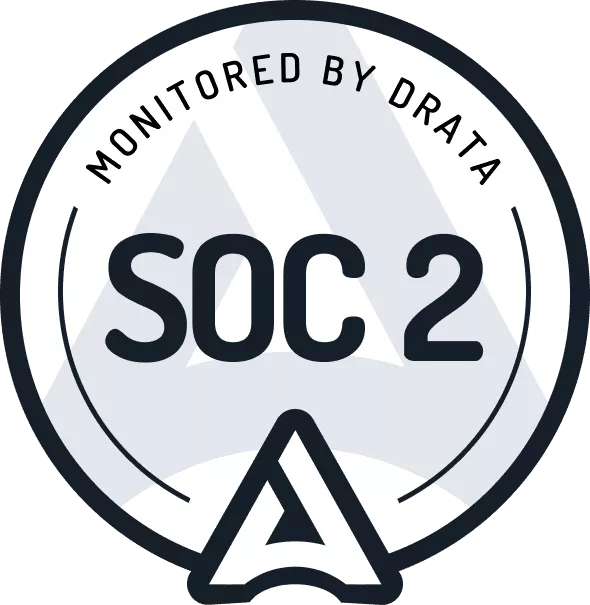# How to Calculate a Finance Lease under ASC 842

## What is a finance lease?

Under ASC 842, the lessee still must perform a lease classification. Under the new lease accounting standard ASC 842, the lease is either an operating lease or a finance lease. A finance lease supersedes a capital lease under ASC 840. Unlike operating leases under ASC 842, accounting for a finance lease under ASC 842 is not too dissimilar to the accounting for a capital lease under ASC 840.

Suppose you've already read our article on how to account for an operating lease under ASC 842. In that case, you will notice in this article that the accounting between an operating lease and a finance lease is very similar. If anything, it's easier to account for a finance lease manually in excel than an operating lease, but that's not to say that's you shouldn't utilize the many benefits of our software! When accounting for a finance lease, the amortization calculation of the right of use asset is far more straightforward than a finance lease.

But before we get ahead of ourselves, the first step is to determine if the lease is a finance lease under ASC 842. When transitioning to ASC 842, the standard setters have a practical expedient that allows companies to grandfather previous lease classifications. As a result, if it's a capital lease under ASC 840, it's a finance lease under ASC 842. For more information on this practical expedient, refer here.

If it's a new lease or you don't want to apply the practical expedient offered. In that case, we have extensive material here to help determine the lease classification.

## Accounting for a capital lease under ASC 840

Under ASC 840 when the lease was classified as a capital lease a lessee was prescribed the following accounting treatment:

ASC 840-30-30:

The lessee shall measure a capital lease asset and capital lease obligation initially at an amount equal to the present value at the beginning of the lease term of minimum lease payments during the lease term

Under ASC 842 the same measurement principle applies under ASC 842.

## Accounting for a finance lease under ASC 842

Under ASC 842, the lessee no longer recognizes a capital lease asset and capital lease obligation but a right of use asset and a lease liability. For finance leases, a lessee is required to do the following:

1. Recognize a right-of-use asset and a lease liability, initially measured at the present value of the lease payments, in the statement of financial position

2. Recognize interest on the lease liability separately from amortization of the right-of-use asset in the statement of comprehensive income

3. Classify repayments of the principal portion of the lease liability within financing activities and payments of interest on the lease liability and variable lease payments within operating activities in the statement of cash flows.

So the key input to the future lease payments has not changed. If you want in-depth analysis, refer to our guide, which covers how the lease liability is measured and how the right of use asset's value is determined.

## Difference between a finance lease and an operating lease under ASC 842

The calculation of the lease liability follows identical principles. The divergence occurs when calculating the "amortization" of the right of use asset. Under an operating lease, a different methodology is used. For more details on how to calculate an operating lease, refer here. Furthermore, under an operating lease, the amortization expense is classified as a lease expense.

The same goes for interest expense. The interest expense for a operating lease is also classified as a lease expense, but the calculation follows the identical methodology as a finance lease.

This has a flow-on impact on a company's cash flow statement. For an operating lease as all cash outflows are classified as "operating" in the statement of cash flows. Furthermore, most debt covenants calculations exclude operating leases as debt.

## Modification accounting for a finance lease under ASC 842

There are many nuances when accounting for modification lease terms under ASC 842. Specific modifications will result in a new lease. All other modifications will trigger a re-measurement of the lease liability and right of use asset. If the modification is a partial termination/decrease in scope, that can also result in an income statement impact.

Refer here for more guidance on if the modification results in a new lease. If you are unsure if the lease is a partial termination, there is more information here and some practical examples of re-measure the lease liability and right of use asset.

## Practical Examples

So it should be clear that a finance lease is not too dissimilar to a capital lease. Everything is already on the balance sheet to begin with. You should also be aware that the lease liability is essentially the present value of known future lease payments. That is the starting point for the right of use asset valuation with some additional inputs, if applicable.

This will be an in-depth calculation example to illustrate how to account for the lease liability and right of use asset to handle any lease permutations, whether that be payments or modifications.

Some areas to note in the calculation methodology are:

• XNPV Microsoft excel formula will be used: this ensures the most accurate calculation of the present as it’s based on the actual timing of payments instead of an NPV formula, which does not. For more details on present value formulas, refer here
• The calculations will be daily: When done manually, most lease calculations are done on a monthly basis using this methodology. You cannot accurately calculate modifications that occur mid-month or ad hoc lease payments accurately.

If you would like the excel calculation of the following examples, please reach out to [email protected].

## Example 1 - Initial Recognition of the right of use asset and lease liability

• Start date: 2021-1-1
• Accounting end date: 2021-1-1(✧)
• Right of use asset end date: 2021-1-1
• Fixed payment amount: \$10,000
• Payment Frequency: Monthly
• Lease Classification: Finance (✧)
• Discount Rate: 7% (✧)
• Right of use asset end date: 2021-12-31

✧: These are the critical judgemental inputs when calculating the lease liability:

• The accounting end date is the most reasonably likely end date of the lease. This may include options to renew the lease if the lessee intends on exercising those options.
• There are several inputs when determining the discount rate. Refer here for more details.
• To determine if the lease is a finance or operating lease, refer here.

### Step 1 - Work out future lease payments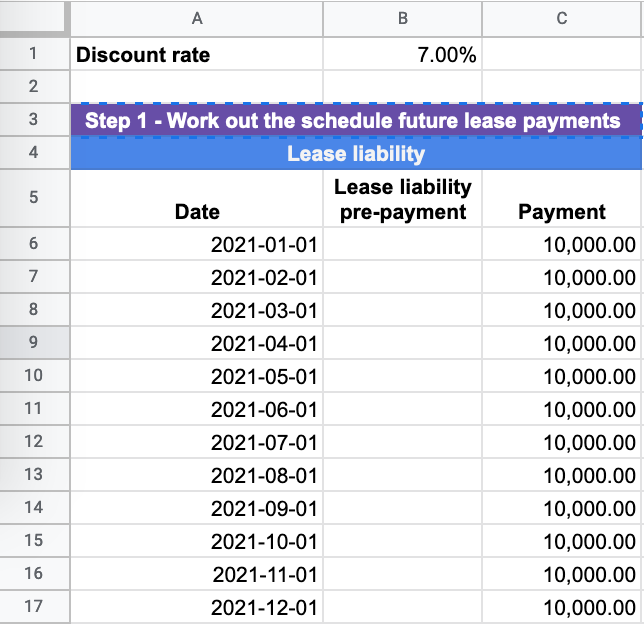When calculating the lease liability, the first step is to work out the known future payments at the start of the lease. In this example, it is 12 payments of \$10,000 occurring on the first day of each month starting on 2021-1-1 to 2021-12-1. Once these payments are present valued, this will be the value of the lease liability.

If you would like more information on what payments should be included in the present value calculation for a finance lease, refer here.

### Step 2 - Determine the discount rate and calculate the lease liability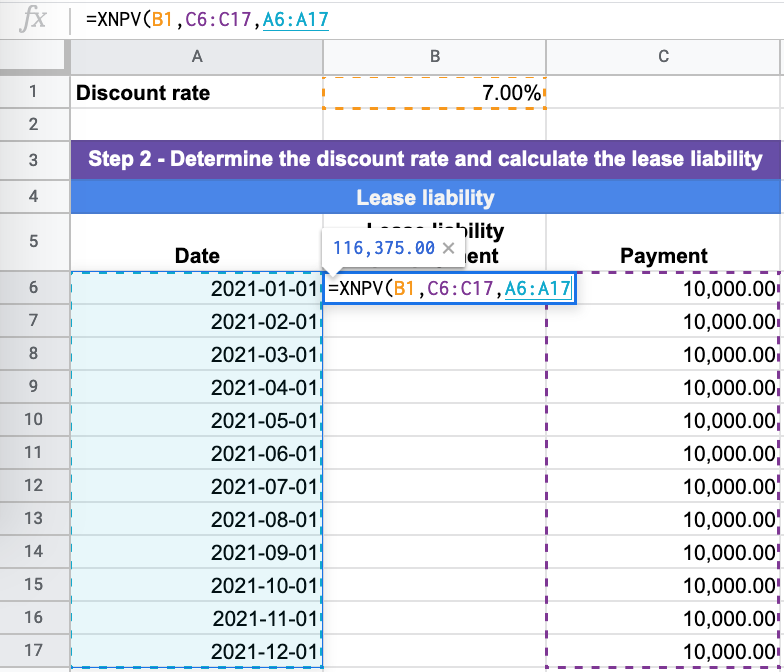The discount rate input into the calculation is one of the most subjective areas when calculating the lease liability. The higher the discount rate, the lower the value of the lease liability at initial recognition. So when you have the discount rate, you have the rest of the inputs to complete the XNPV calculation, which are:

• Cash Flow amounts
• Cash Flow dates

In this example, the present value at 2021-1-1 of the lease liability is \$116,375. This is the amount that will be recognized on the balance sheet. If you would like further information on how to determine the discount rate refer here. If you would like greater detail on the concept of present valuing and the different options available, refer here.

### Step 3 - Calculate the right of use asset value

Without the value of the lease liability, you cannot calculate the right of use asset. In this example, there are no other inputs that will impact the value of the right of use asset. As a result, in this example, the value of the right of use asset will be \$116,375, the same amount as the lease liability.

For further information on what inputs can impact the value of the right of use asst refer here.

### Step 4 - Calculate the unwinding of the lease liability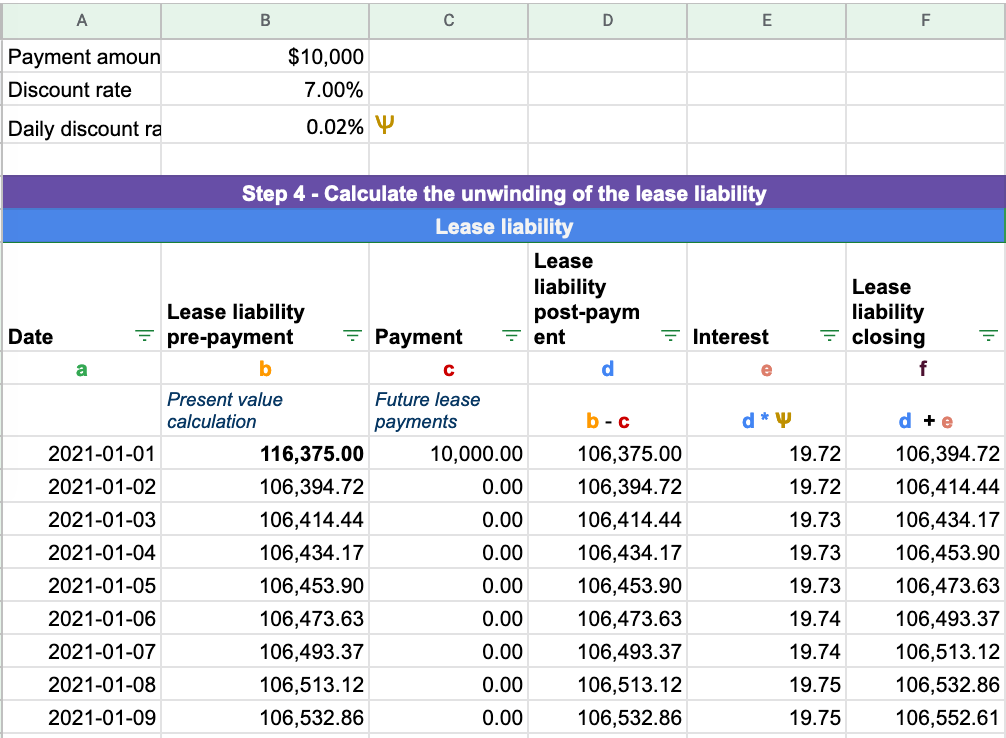Once you have the lease liability's present value, the next step is to calculate the lease liability's unwinding over time. As payments are made, the lease liability should ultimately unwind to \$0. We'll break down the calculation in reference to the picture above. The formulas input do not change row by row.

Column A - Date: This column captures every day applicable to the agreement. In this case, it’s 2021-1-1 to 2021-12-31. Drag the cell down, and the days will populate.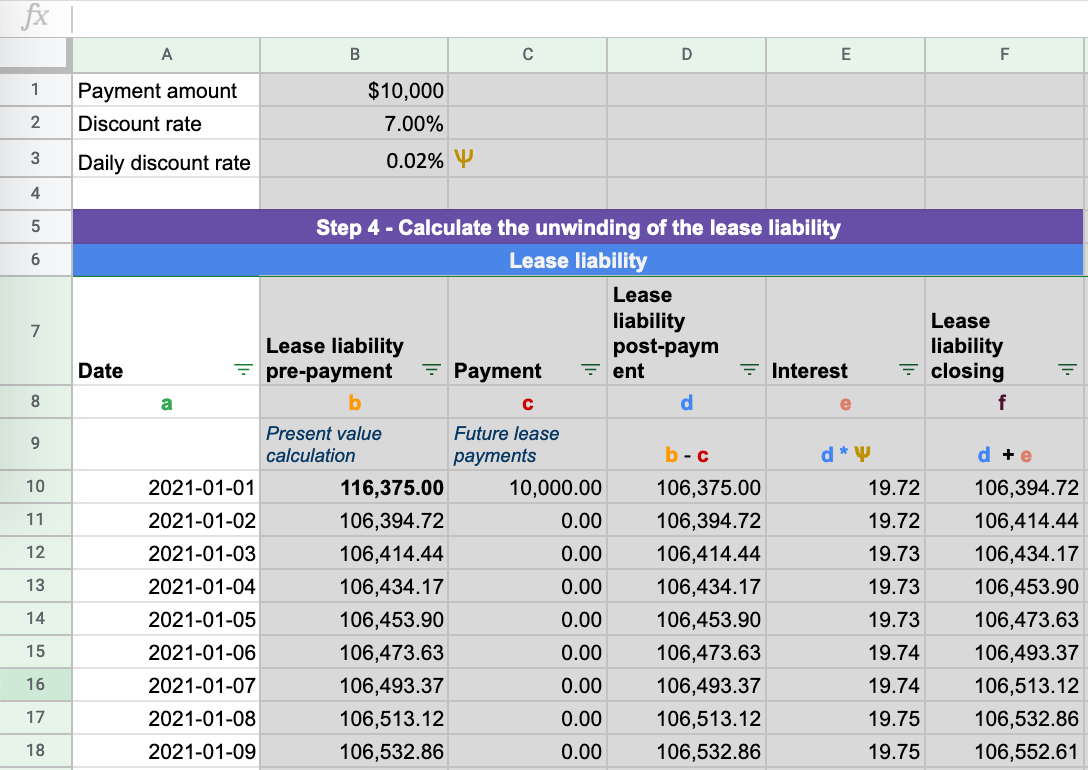Column B - Lease liability prepayment - Where the present value XNPV formula is input for each row: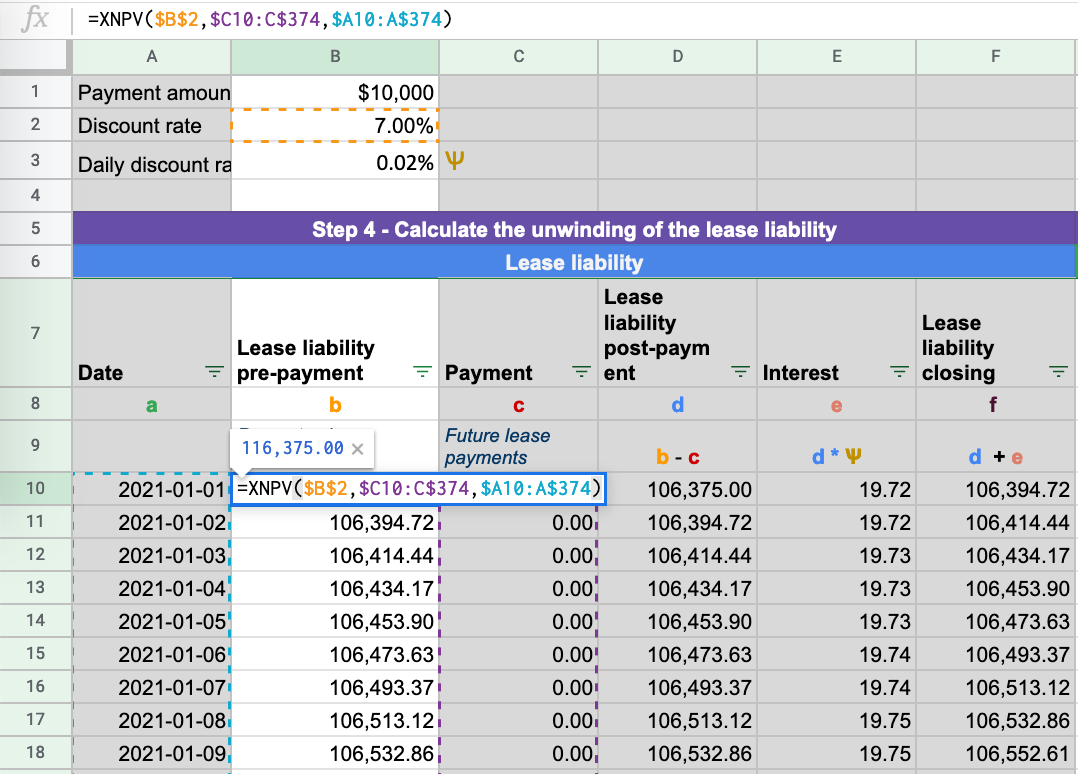Column C - Payment - Future lease payments at each particular date: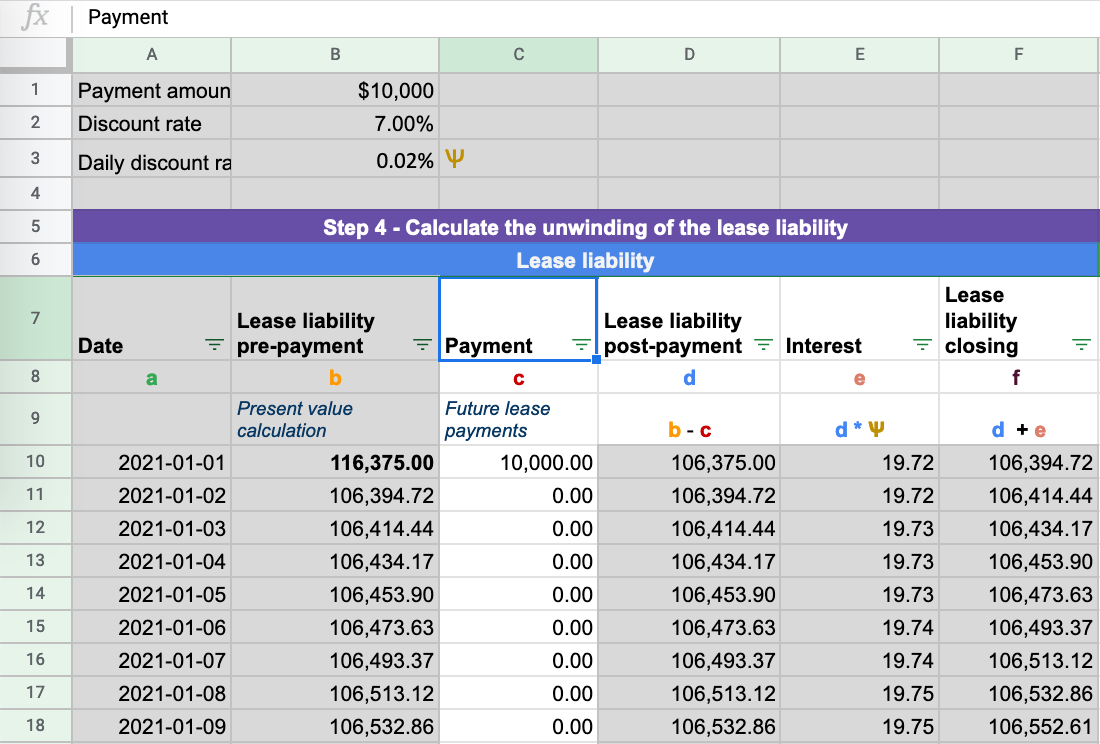Column D - Lease liability post-payment - This is the lease liability amount post-payment. Payments reduce the lease liability balance: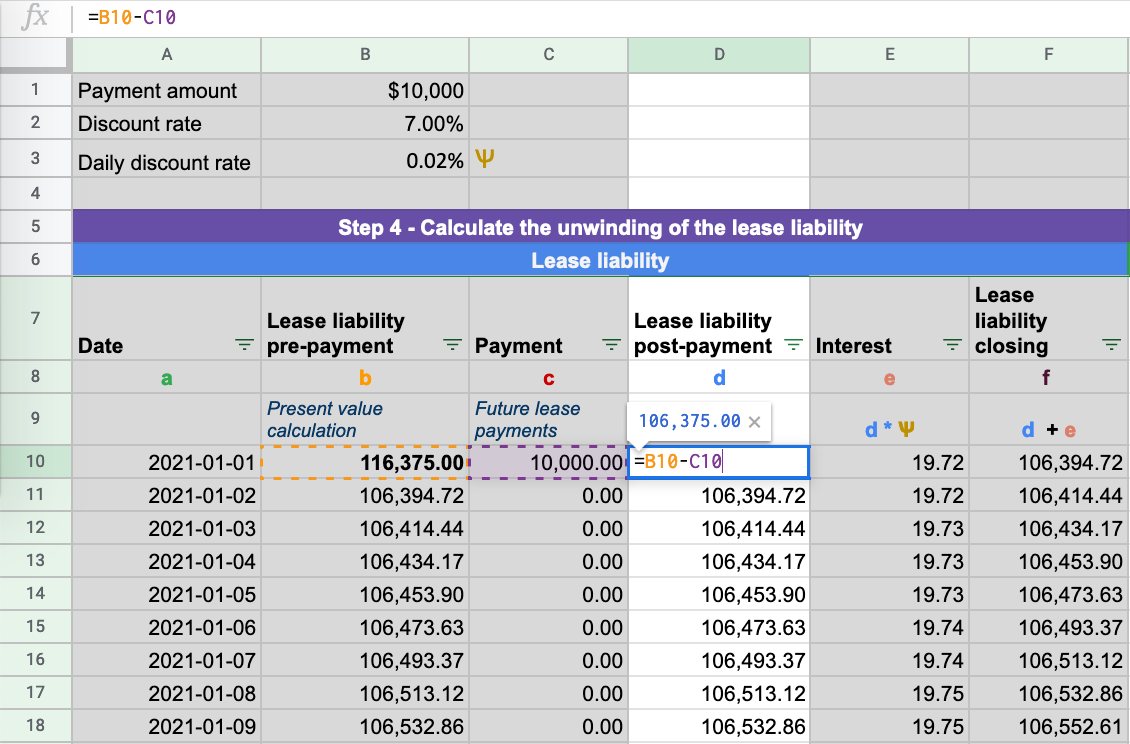Column E - Interest - This is the daily interest amount calculated on the lease liability based on the daily discount rate: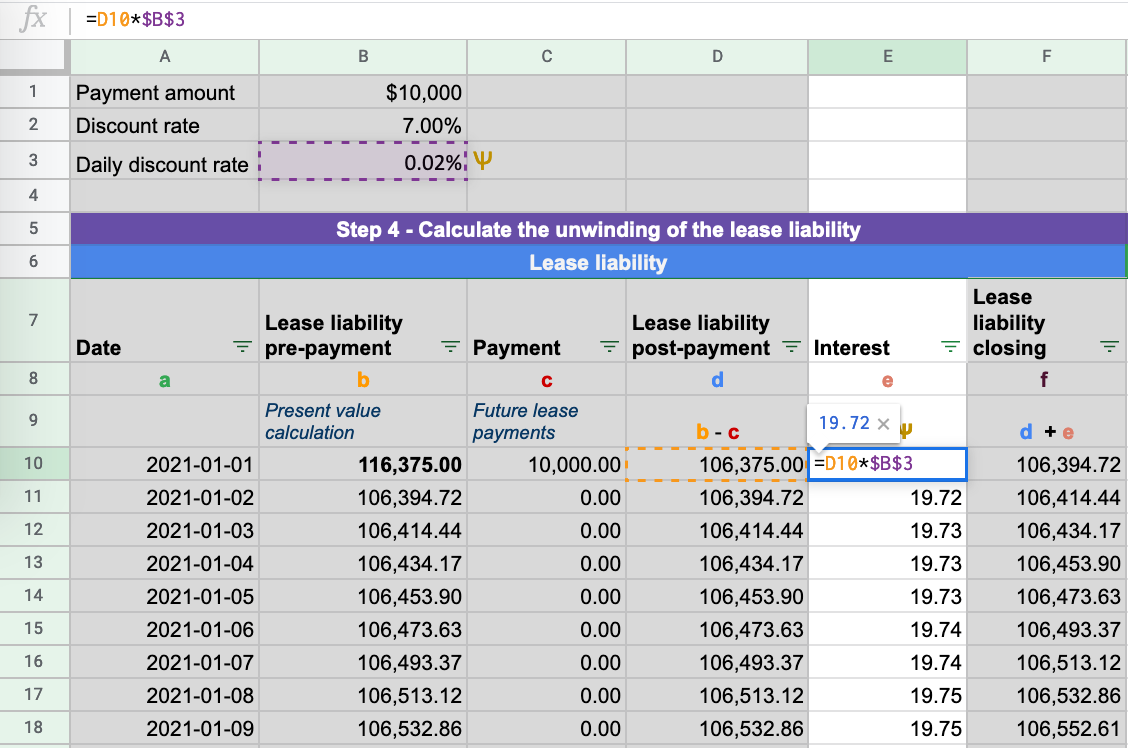Column F - Lease liability closing balance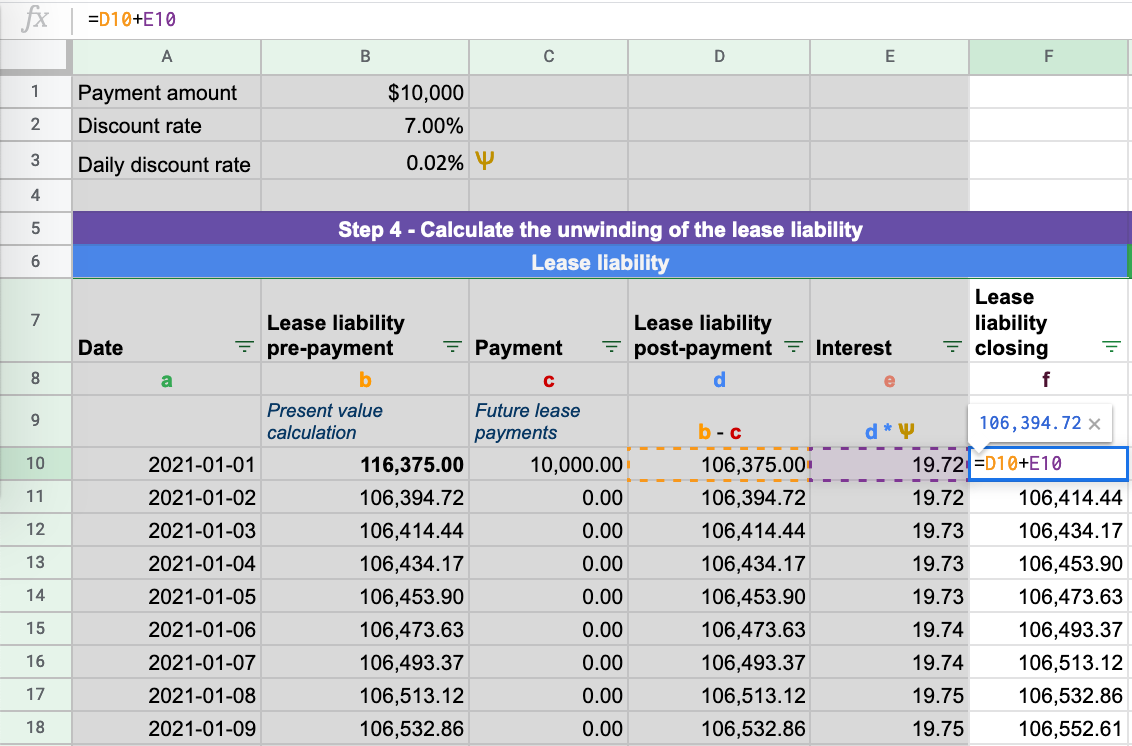To ensure your lease liability has been calculated correctly ensure it unwinds to zero as shown in the below animation: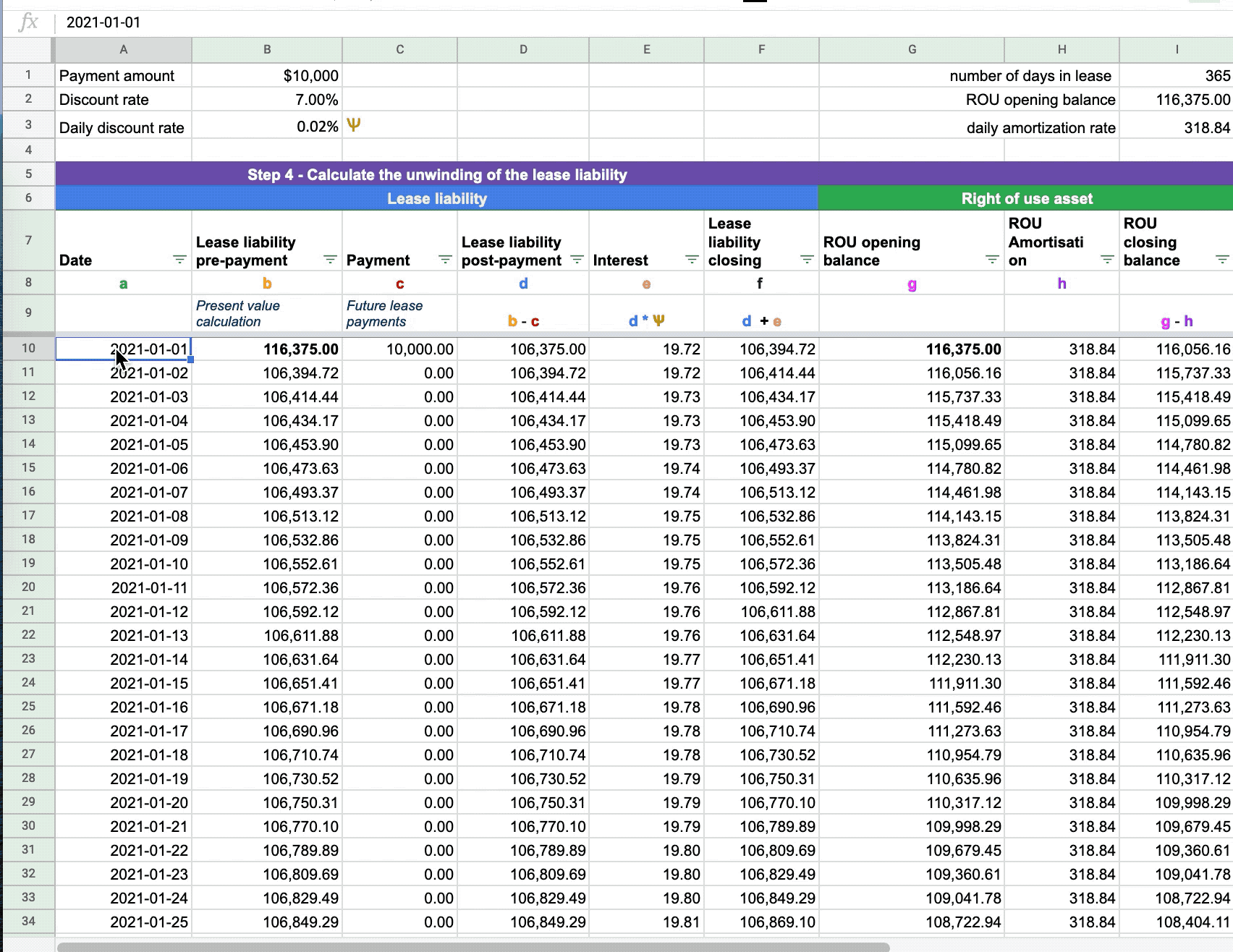### Step 5 - Calculate the right of use asset amortization rate

Given there have been no other inputs to impact the value of the right of use asset. The opening value is equal to the lease liability value. For further information on how to calculate the initial recognition balance, refer here. In this example, the right of use asset value is 116,375.00.

The amortization for a finance lease under ASC 842 is very straightforward. To calculate the straight-line amortization is the opening value of the right of use asset divided by the number of days of the useful life.

Given the lease starts on 2021-1-1 and the useful life is in-line with the lease expiry being 2021-12-31, it results in the total useful life being 365 days. The right of use asset opening value is 116,375. The calculation is then 116,375 / 365 = \$318.84.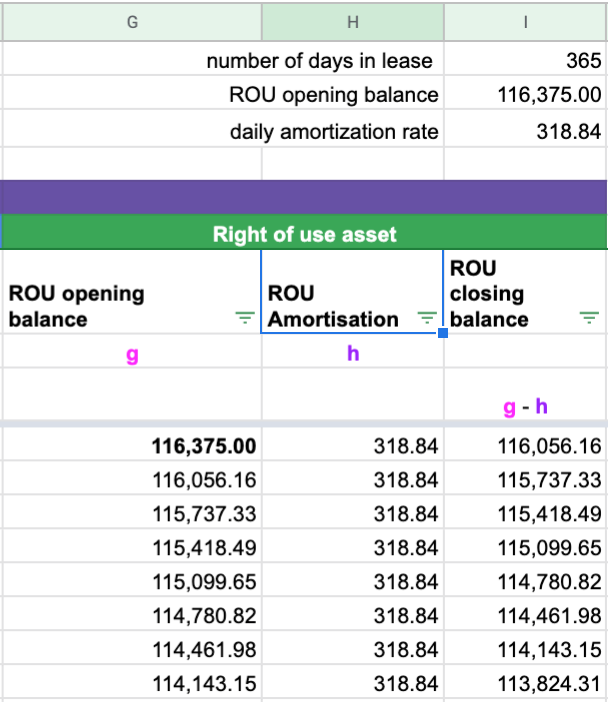Once you have updated the formulas until 2021-12-31, the finance lease calculation under ASC 842 is complete. You will derive the month to month journal entries from these calculated amounts, assuming there are no modifications. This spreadsheet will also be used for many of the quantitative disclosure requirements of the standard.

## Example 2 Scenario - Modification Accounting

Example 2 will display the necessary calculations for a specific modification for a finance lease under ASC 842. When a modification occurs, it's either going to impact the payment amount or the lease's length and sometimes both. As you've probably realized, these are the two major inputs when calculating the future lease payments that are then present valued to form the lease liability for a finance lease under ASC 842.

We'll now go through the following calculation steps of a modification that increases the fixed payments for a finance lease under ASC 842.

Using the details of Example 1:

•     Start date: 2021-1-1
•     Accounting End date: 2021-12-31
•     Fixed Payment Amount: \$10,000
•     Payment Frequency: Monthly
•     Lease classification: Finance Lease
•     Discount rate: 7.00%

Modification to the terms:

•     On 2021-10-16, the fixed payment amount increased to \$12,000 for the remaining lease payments
•     The appropriate discount rate at modification is 6.00%

If you would like the excel calculation for Example 2, please reach out to [email protected].

### Step 1 - Work out the modified future lease payments

In this example’s modification, it’s the future lease payments that have been modified. The lease length does not change.  The remeasurement of the lease liability and right of use asset will occur on October 16, 2021, with the contractual future cash flows on 2021-11-1 and 2021-12-1 being modified from \$10,000 to \$12,000.  As a result, the payments now like this: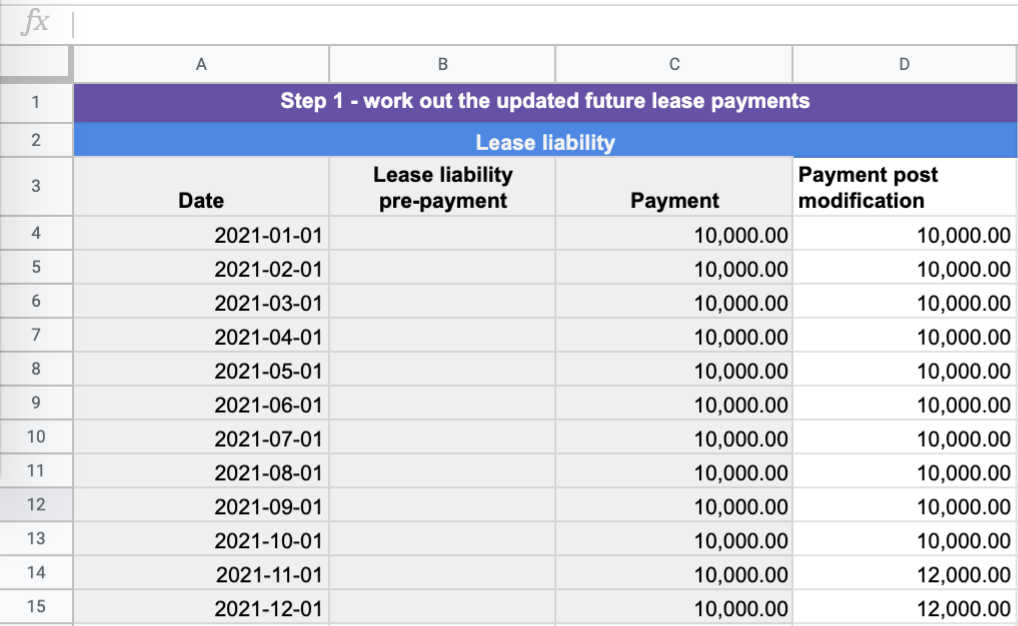### Step 2 - Determine the appropriate discount rate and re-calculate the lease liability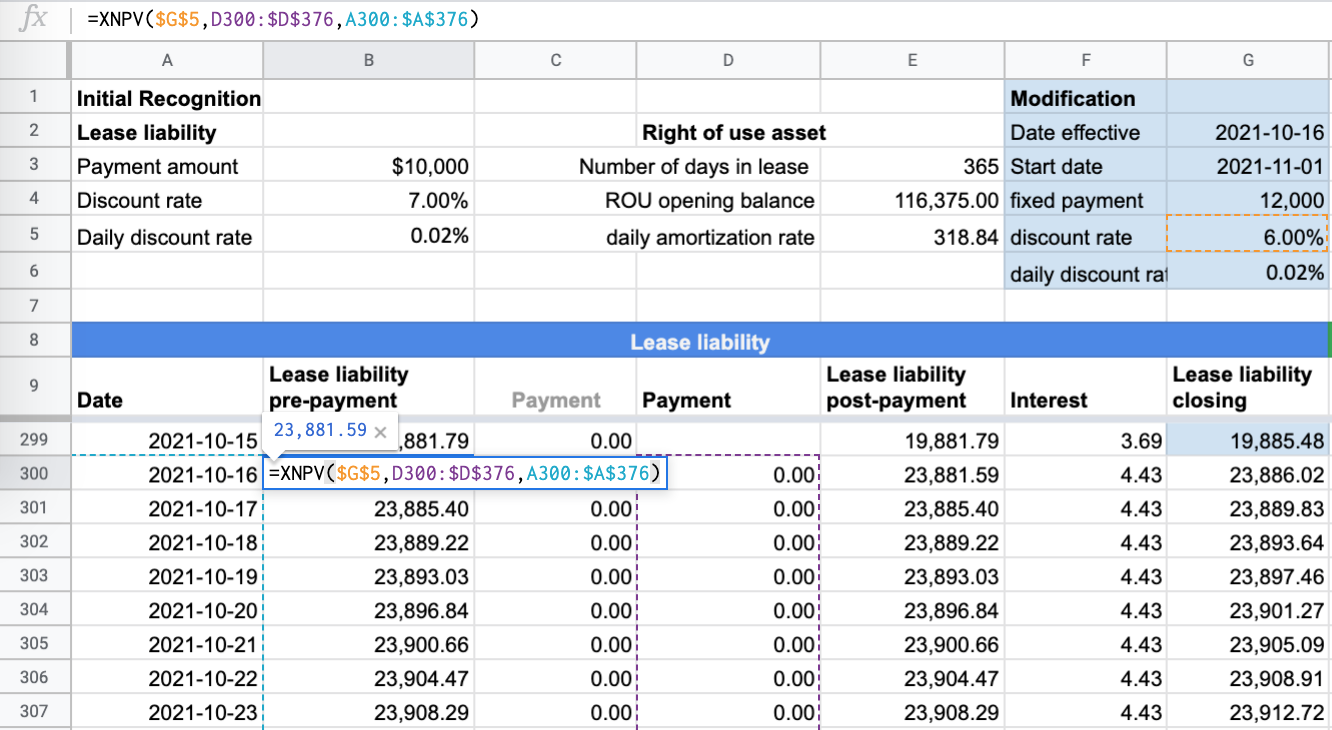In relation to Example 2, the following have changed in relation to the calculation on 2020-10-16:

• An updated discount rate of 6% in CELL G5
• Values: The XNPV formula values will present value the updated future payments of Column D.

Based on adding a new column the following updates will need to be made to the calculation:

a) Lease liability post payment will subtract payments from column D as opposed to column C: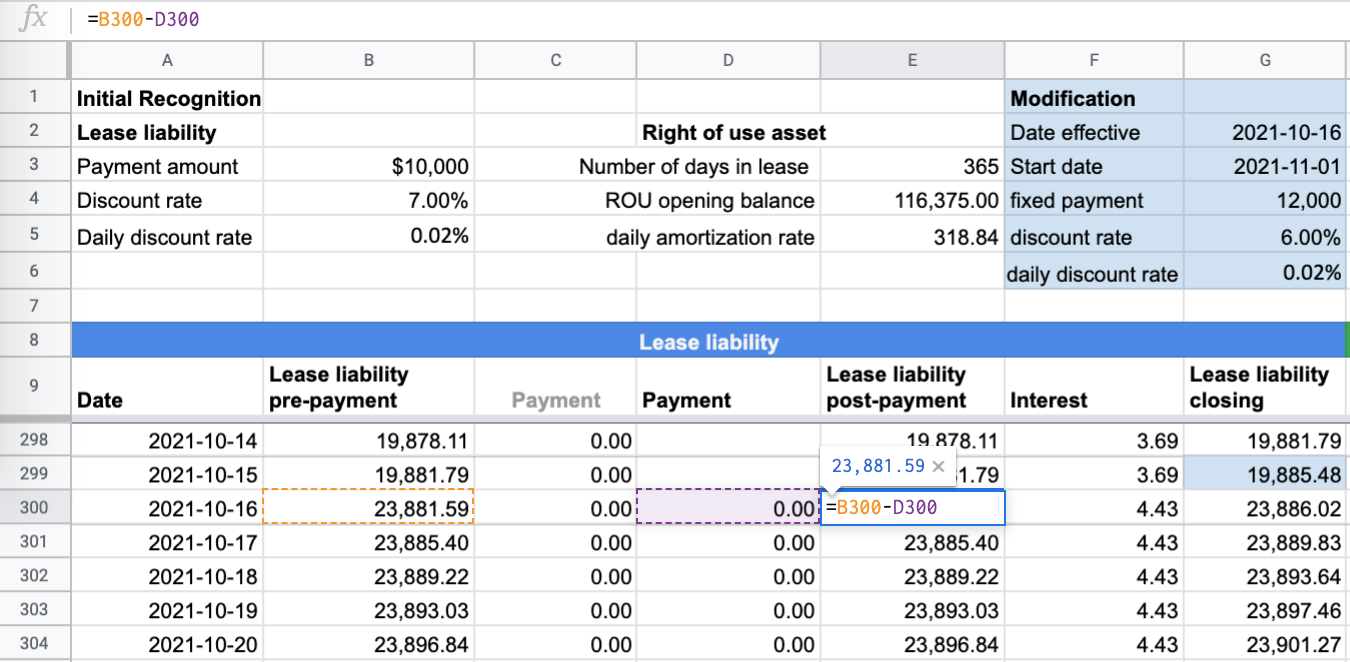b) Daily interest calculation will use the updated daily discount rate: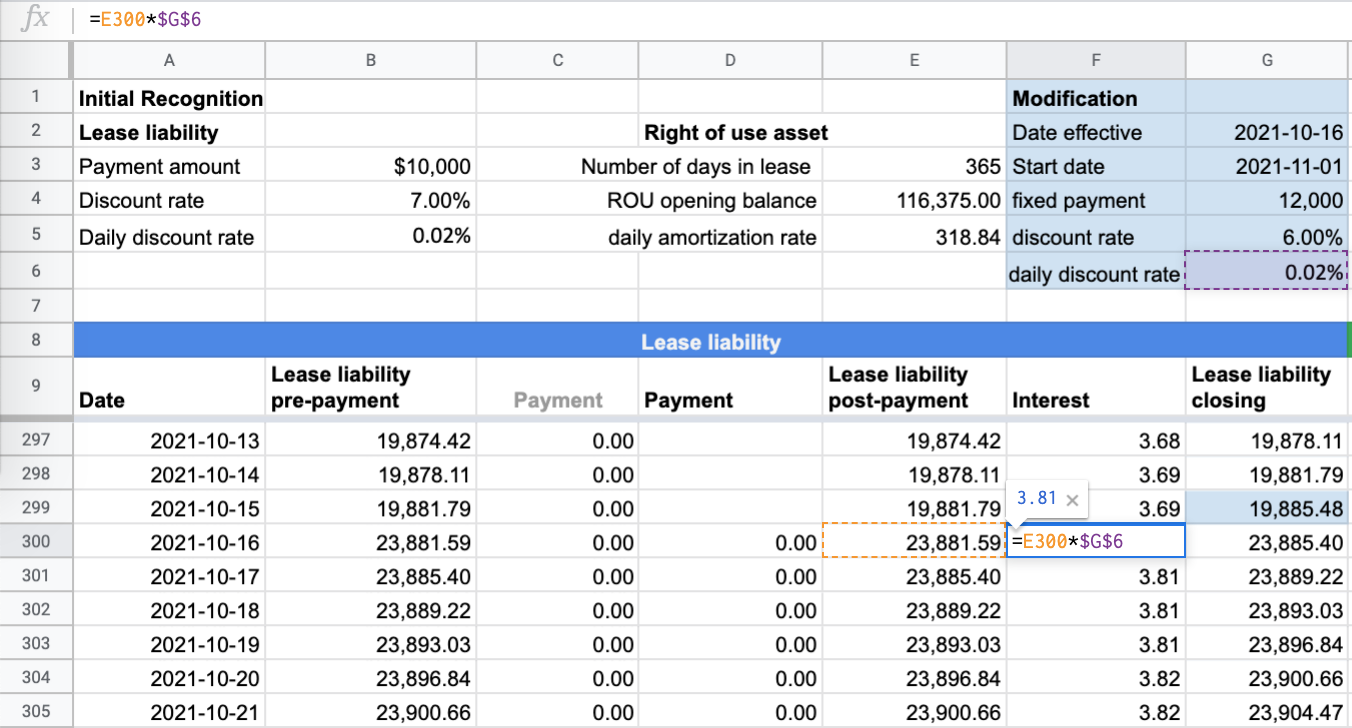When a modification occurs, ASC 842 prescribes a company to use an updated discount rate. In the case of Example 2, the discount rate has gone from 7% to 6%. This will then have an impact on the daily discount rate used to calculate the daily interest charge. From a calculation perspective, you also need to remember to update the calculation to use the latest discount rate in the XNPV formula and the calculation of interest earned on the lease liability.

c) Ensure the lase liability correctly unwinds to \$0 with the updated formulas for each row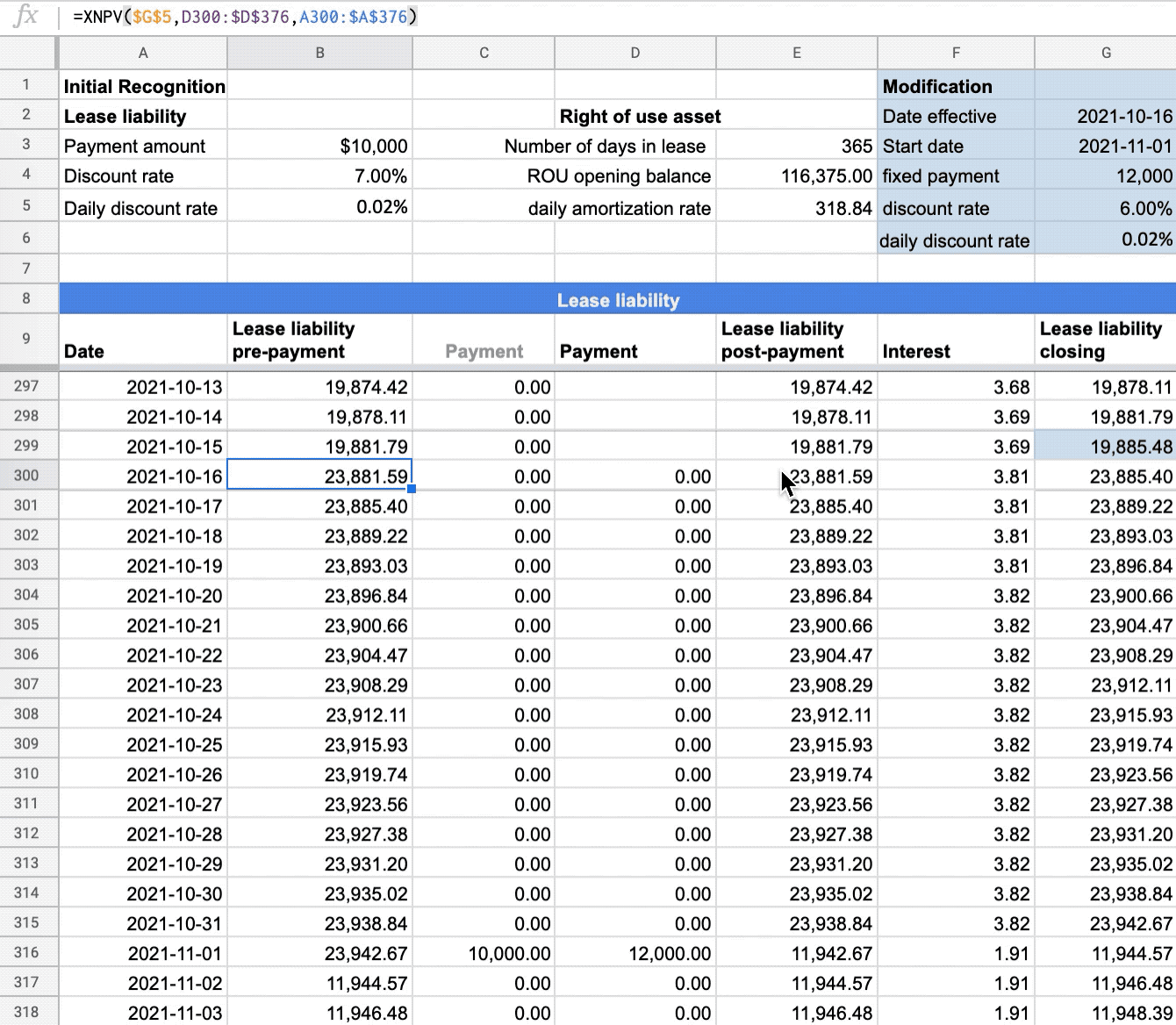### Step 3 - Capture the modification movement and apply that to the ROU asset value

Because there has been a change to the lease liability value, and in this case, the value has increased, it results in a credit to the balance given it’s a liability. So what is the other side of the journal entry? The debit must go somewhere. The standard prescribes that the amount goes to the right of use of the asset.

To work out this value, you must compare the lease liability before modification and then the value post-modification. This will give you the remeasurement amount. With Example 2, the lease liability amount before modification was \$19,885.48. This figure is the closing balance at 2021-10-15 or the opening balance of 2021-10-16. The post-modification value of the lease liability value is \$23,881.59. This results in a movement of \$3,996.11. The remeasurement journal entry is then:

Dr Right of use asset \$3,996.11

Cr Lease liability \$3,996.11

The closing balance of right of use asset value at 2021-10-15 is \$24,550.34. The addition of \$3,996.11 results in the right of use asset value post modification of \$28,546.45 (\$24,550.34+\$3,996.11) at 2021-10-16 before amortization.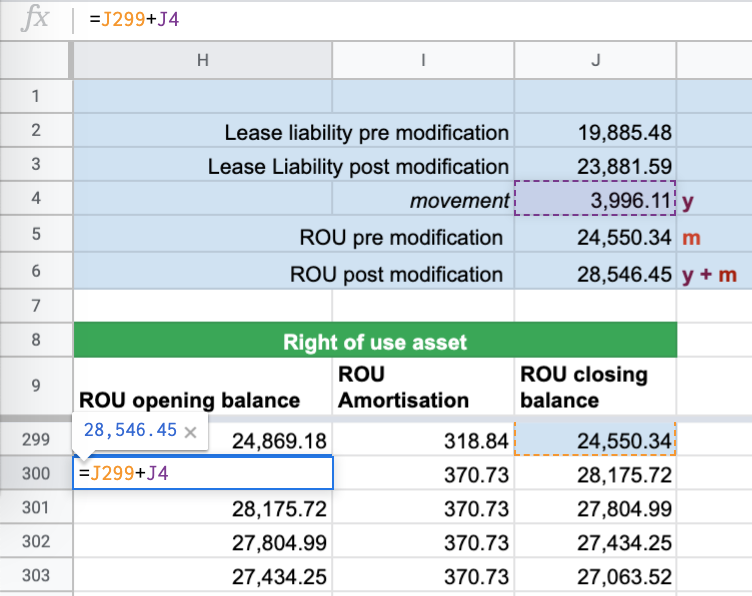### Step 4 - Update the right of use asset  amortization rate

Given the right of use asset value has changed, the pre-modification amortization rate is now incorrect. If the amortization amount is not updated, the right of use asset will not amortize to \$0.

With a finance lease under ASC 842, the calculation methodology to calculate the amortization rate post modification follows the same methodology at initial recognition. Value of the right of use asset divided by total remaining useful life days. As a result the calculation will be \$28,546.45 / 77 = \$370.73.

To ensure you’ve calculated the correct amortization amount make sure the right of use asset is \$0 at 2021-12-31.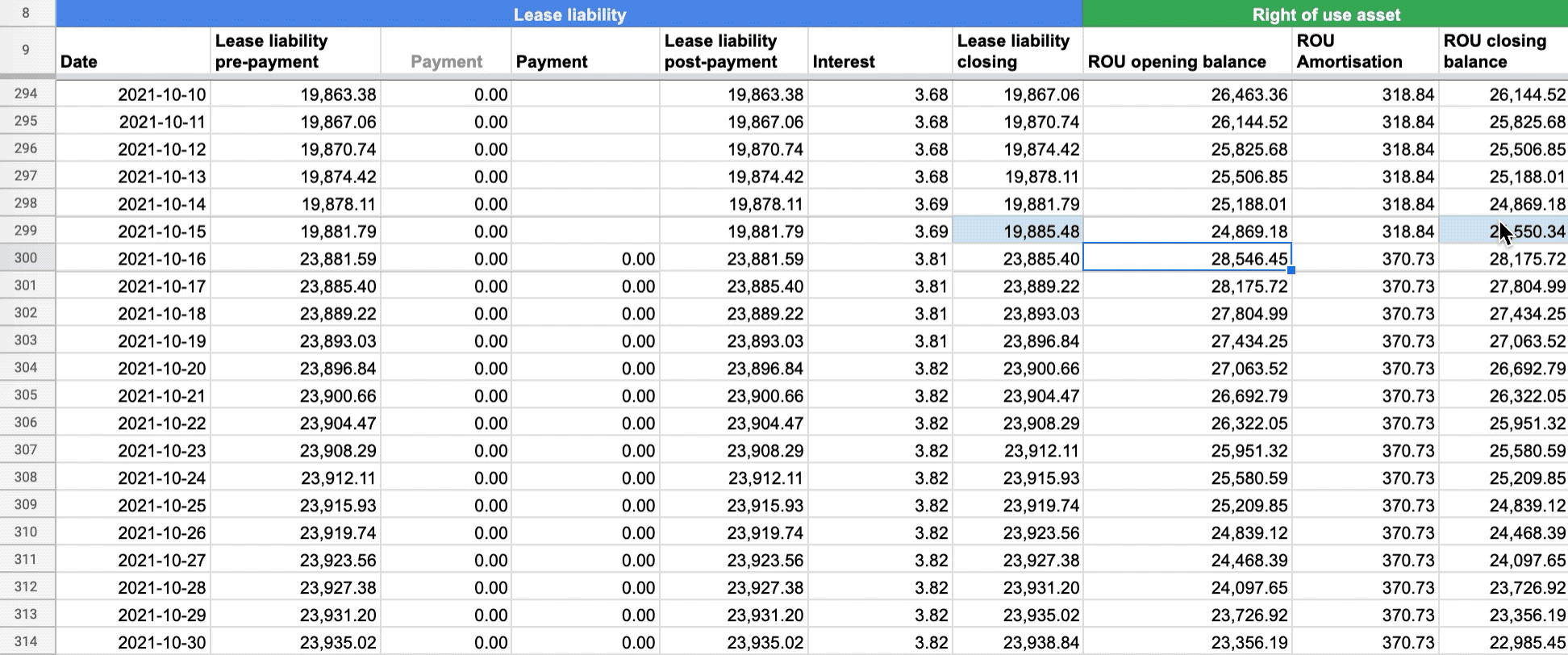Once you have completed these steps, your calculation has been updated to ensure compliance for a finance lease under ASC 842. From this spreadsheet, you can derive the correct journals from now on.

## FooterHere at Cradle, our mission is simple; it's at the foundation of everything that we do. We want to make accountants' lives easier by leveraging technology to free up their time to focus on running the business.

140 Yonge St.
Suite 200
Toronto, ON M5C 1X6

US
Suite #73591
Torrance, CA 90503
USA

### Legal